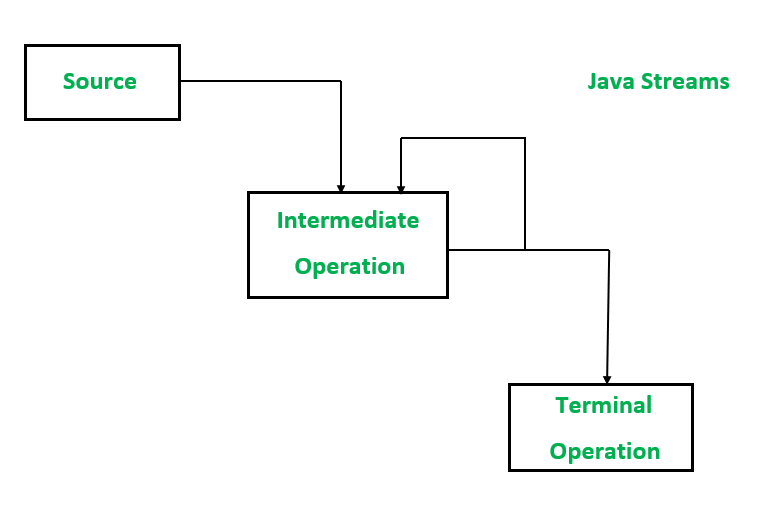Open in App
Not now

# Functional Programming in Java 8+ using the Stream API with Example

• Difficulty Level : Easy
• Last Updated : 09 Dec, 2021

API is an acronym for Application Programming Interface, which is software and the java streams work on a data source. Consider a stream like a flow of water in a small canal. Let’s take a real-life example. Each time a user uses an application that is popular these days like WhatsApp in order to communicate via delivering text messages or calls to other users. Both users are using an API.Java streams work on three operations which as mentioned below

1. Data Source
2. Intermediate operation
3. Terminal operation

Methods: Streams can be created in three ways

1. Using an object of any class from the collection framework
2. Using an array of the reference data type
3. Using the interface defined in the ‘java.util.stream’ package

Method 1: Data Source

The data source can be widely varied such as an array, List, etc

Syntax:

```ArrayList<Integer> numbers = new ArrayList<>();
Integer[] numbers = {1,2,3};```

Example 1: Using an object as a data source

## Java

 `// Java Program showcasing data source``// using an object as a data source` `// Importing input output classes``import` `java.io.*;` `// Class``class` `GFG {` `    ``// Main driver method``    ``public` `static` `void` `main(String[] args)``    ``{``        ``// Data Source` `        ``// Creating an arrayList object``        ``// Declaring object of String type``        ``ArrayList gfgNames = ``new` `ArrayList<>();` `        ``// Custom input elements to above object``        ``gfgNames.add(``"Dean"``);``        ``gfgNames.add(``"castee"``);``        ``gfgNames.add(``"robert"``);` `        ``// Creating object of Stream where Stream is created``        ``// using arrayList and object as data source``        ``Stream streamOfNames = gfgNames.stream();` `        ``// Print and display element``        ``System.out.print(streamOfNames);``    ``}``}`

Example 2: Using an array as a data source

```// Data Source
Integer[] numbers = {1,2,3,4,5};```
```// Stream using an array
Stream<Integer> streamOfNumbers = Arrays.stream(numbers);```
```// using predefined Instream interface
integerStream = IntStream.range(1,100); // a stream from 1 to 99;```

## Java

 `// Java Program showcasing data source``// using an array as a data source` `// Importing java input output class``import` `java.io.*;` `// Importing all classes from``// java.util package``import` `java.util.*;` `// Importing class for additional operations,``// additionls and pipelines``import` `java.util.stream.IntStream;` `// Class``class` `GFG {` `    ``// Main driver method``    ``public` `static` `void` `main(String[] args)``    ``{``        ``// Creating a predefined stream using range method``        ``// Custom inputs for range as parameters``        ``var stream = IntStream.range(``1``, ``100``);` `        ``// Stream is created``        ``var max = stream.filter(number -> number % ``4` `== ``0``)``                      ``.count();` `        ``// Print and display the maximum number``        ``// from the stream``        ``System.out.println(max);``    ``}``}`

`24`

Method 2: Intermediate Operation

Intermediate operations are some methods that one can apply on a stream.

Note: It can be of any number

`filter()`

Example:

## Java

 `import` `java.io.*;` `class` `GFG {``    ``public` `static` `void` `main(String[] args)``    ``{``        ``// Data Source``        ``Integer[] numbers = { ``1``, ``2``, ``3``, ``4``, ``5``, ``6``, ``7``, ``8``, ``9``, ``0` `}` `        ``// Stream``        ``Stream streamOfNumbers``            ``= Arrays.stream(numbers);` `        ``// filter all the even numbers``        ``Stream evenNumbersStream``            ``= streamOfNumbers.filter(``                ``number -> number % ``2` `== ``0``)``    ``}``}`

Method 3: Terminal operation

Terminal operation methods that we can apply on a stream that will cause a stream to be “closed”.

Concept:

Some terminal operations can be used to iterate on the elements of the stream.

`min(),max(),count()`
`forEach(),noneMatch()`

Example 1: Explaining stream API

## Java

 `// Importing input output classes``import` `java.io.*;``// Importing all classes from``// java.util package``import` `java.util.*;` `// Class``class` `GFG {` `    ``// Main driver method``    ``public` `static` `void` `main(String[] args)``    ``{``        ``// Data source``        ``// Custom integer inputs``        ``Integer[] numbers``            ``= { ``1``, ``2``, ``3``, ``4``, ``5``, ``6``, ``7``, ``8``, ``9``, ``0` `};` `        ``// Stream``        ``var streamOfNumbers = Arrays.stream(numbers);` `        ``// Using filter method``        ``var evenNumbersStream``            ``= streamOfNumbers``                  ``.filter(number -> number % ``3` `== ``0``)``                  ``.count();` `        ``// Print and display``        ``System.out.println(evenNumbersStream);``    ``}``}`

Output

`4`

Example 2:

## Java

 `// Importing all classes from java.util package``import` `java.io.*;``import` `java.util.*;` `// Class``class` `GFG {` `  ``// Main driver method``    ``public` `static` `void` `main(String[] args)``    ``{``      ``// Creating an ArrayList of Integer type``        ``ArrayList list = ``new` `ArrayList<>();``      ` `      ``// Adding elements to above object of Arraylist``      ``// Custom inputs``        ``list.add(``20``);``        ``list.add(``4``);``        ``list.add(``76``);``        ``list.add(``21``);``        ``list.add(``3``);``        ``list.add(``80``);` `        ``var stream = list.stream();` `        ``var numbers``            ``= stream.filter(number -> number % ``2` `== ``0``)``                  ``.filter(number -> number > ``20``);``      ` `      ``// Print all the elements of the stream on the console``        ``numbers.forEach(System.out::println);``    ``}``}`

Output

```76
80```

Note: One can pass lambda also number -> System.out.println(number + ” “)

My Personal Notes arrow_drop_up